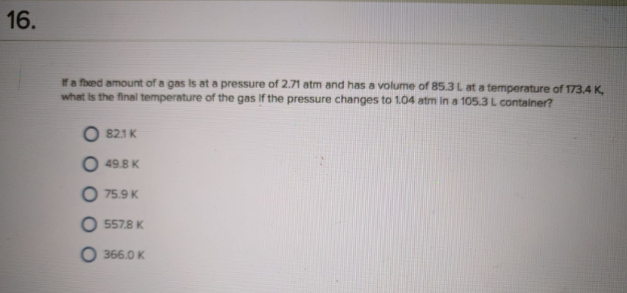# Problem: If a fixed amount of a gas is at a pressure of 2.71 atm and has a volume of 85.3 L at a temperature of 173.4 K, what is the final temperature of the gas if the pressure changes to 1.04 atm in a 105.3 L container?a. 82.1 Kb. 49.8 Kc. 75.9 Kd. 557.8 Ke. 366.0 K

###### FREE Expert Solution
90% (190 ratings)###### Problem Details

If a fixed amount of a gas is at a pressure of 2.71 atm and has a volume of 85.3 L at a temperature of 173.4 K, what is the final temperature of the gas if the pressure changes to 1.04 atm in a 105.3 L container?

a. 82.1 K

b. 49.8 K

c. 75.9 K

d. 557.8 K

e. 366.0 K